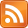# The Derivative of a Procedure

20 Jan 2014, 09:48 UTC

So this is pretty out there even for me. Be warned!

Suppose you have a procedure which takes a set, as follows:

``````void proc(set s)
{
for (a in s) {
CreateSomeFile(a.name) << "Hello world\n";
}
}
``````

If we give this procedure a set of objects with names "foo.txt" and "bar.txt", it will create two text files. If we give this procedure a set of 10,000 objects it will create 10,000 text files. But if we then add one object to the set and wish to run the procedure again, we'll duplicate work 10,000 times just to create one file.

In that case we'd probably refactor our application to work like this:

``````void Init(set s) { proc(s); }

{
CreateSomeFile(ds.name) << "Hello world\n";
}
``````

Now here's the weird bit: OnObjectAdded is the derivative with respect to s of proc. Wha?

Well if you have a function f(x), then the derivative is df/dx. This means if you step along x by some dx, then f(x + dx) ~= f(x) + df/dx * dx. The term df/dx * dx might be considered a function of dx so f(x + dx) ~= f(x) + g(dx) where g(dx) = df/dx * dx is effectively "the derivative of f(x)". (In fact df/fx might be a function of x so we really need g(x,dx) - as we'll see later.)

[Advanced note: f(x + dx) ~= f(x) + g(x, dx) but = f(x) + g(x, dx) + correction(x,dx).]

In our case, if we're working in an additive algebra of side-effects, SideEffects[proc(s+ds)] = SideEffects[proc(s)] + SideEffects[OnObjectAdded(ds)].

Meh, you might say, this is just a weird way to look at it.

But then you discover the chain rule and it starts to seem like we're onto something. Take the new procedure:

``````void proc2(set s)
{
for (a in s) {
CreateSomeFile(a.name) << s;
}
}
``````

``````void OnObjectAdded2(set s, object ds)
{
for (a in s) {
}
CreateSomeFile(ds.name) << (s + ds);
}
``````

Now, recall the chain rule: d/dx[f(x)*g(x)] = df/dx*g(x) + f(x)*dg/dx. This creates two terms in the derivative - like the two parts of our OnObjectAdded2 function. The two parts are f(x) unchanged, but multiplied by dg/dx, and g(x) unchanged, but multiplied by df/dx.

To create our OnObjectAdded2 we considered two things. First, what new files are created, second, what new data is added to the existing files. This came about by looking at what changed in the loop "for (a in s)" and looking at what changed in the contents of the file "<< s". The result has two parts:

1. for (a in s) unchanged, but containing the derivative of the contents of the loop (the derivative of "CreateSomeFile(a.name) << s" wrt s is "AddToFile(a.name) << ds")
2. for (a in s) derived, but containing the unchanged contents of the loop (the derivative of "for (a in s) { ... }" is "{ a = ds; ... }".

So the chain rule for differentiation formalizes the process we go through manually when we write such a function. Weird, huh?!

[Advanced note: we can run 1 and 2 in any order, but in both cases the first thing uses just "s" and the second thing uses "s + ds" ... so in the code above, (s + ds) is added to the new files, not just s, otherwise ds would be missing. This is the "correction(x,dx)" and is equivalent to "AddToFile(ds.name) << ds" - i.e. a 2nd-order term in ds.]

So far this just deals with adding to sets (ds is "positive"); with deleting from sets (ds is "negative") we need more. What we need are "side-effect inverses" of the procedures we call - the equivalent of a "minus sign". The inverse of CreateSomeFile is of course DeleteSomeFile (no "of course" about it - in fact, it's actually "RecoverOldVersionOfFile", but let's play a let on that one for now). The inverse of "AddToFile(x) << ds" has to be "RemoveFromFile(x) << ds" (meaning remove the entry ds from the file, wherever it is in the file). Then we can write:

``````void OnObjectDeleted2(set s, object ds)
{
for (a in s) {
RemoveFromFile(a.name) << ds;
}
DeleteSomeFile(ds.name);
}
``````

### And This is Useful How?

Well, it seems to me this could serve as a useful "bridge" between straightforward imperative code, and more complex "reactive" code. I mean, writing OnObjectAdded and OnObjectDeleted is bread-and-butter programming work. We've all done it so often that it's just maybe never occurred to us that these procedures are mathematically related to the original dumb procedure of just recreating the whole world each time. Suppose we could take any dumb program that assumed a blank slate and did stuff, and transform it into a smarter program that did stuff reactively given a current state? That's like a whole programming idiom, automated.

A smart reader will have noticed that this doesn't work if the "side-effect inverse" doesn't exist or can't be found. Our inverses are "independent" in that removing some element from the file has to be orthogonal to removing a different element from the file, and elements may be added or removed in any order. What if that's not possible? There must surely be some restrictions on the type of side-effect we can handle. But if we could handle any database update, then we might have a quite powerful tool that could take any functional code plus database side-effects and convert it into reactive code, automatically.

Just a thought, anyway.